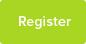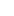# Tutor Hunt Questions

what amount of water would be needed to be added to 10g of ammonium nitrate to change the temperature of an instant cold pack form 20 degrees to 5 degrees? (6 marks)
3 years ago

Please enter your response to the question below. The student will get a notification as soon your response has been approved by our moderation team.

You can calculate the actual answer by the following method:

>>>1. Assume: the change is adiabatic (i.e. very fast or very well insulated the specific heat capacity of the solution is the same as for water (c=4.2kJ/kg/K) that the Enthalpy ("Heat") of Solution for NH4NO3 is +25.8kJ/mol.

>>>2. Find the molar mass of NH4O3: (14+4x1+14+3x16 = 80)

>>>3. Find the no. of moles you have: 10/80 = 1/8

>>>4. Calculate the Change in Heat (dH) as dH = 25.8kJ/mol x 1/8mol = 3.225kJ

>>>5. Realise that dH is also: dH = Ms x c x dT [where Ms is the mass of the solution, c is the specific heat capacity given above and dT is the temperature change (20 - 5 = 15 in your case).

>>>6. Note that Ms = M + Mw (where M is your "10g" or 0.01kg of NH4NO3 and Mw is the mass of water that you are trying to find)...
>>>So: Ms = dH/c.dT

>>>putting the numbers in gives:
Ms = 3.225/(4.2x15 = 0.05kg

>>>>so finally, Mw = Ms - M = 0.05 - 0.01 = 0.04kg or 40g (i.e. 40ml) of water .... the question vaguely asked for "amount" of water so I guess either the mass or volume would do.